# 题目描述（困难难度）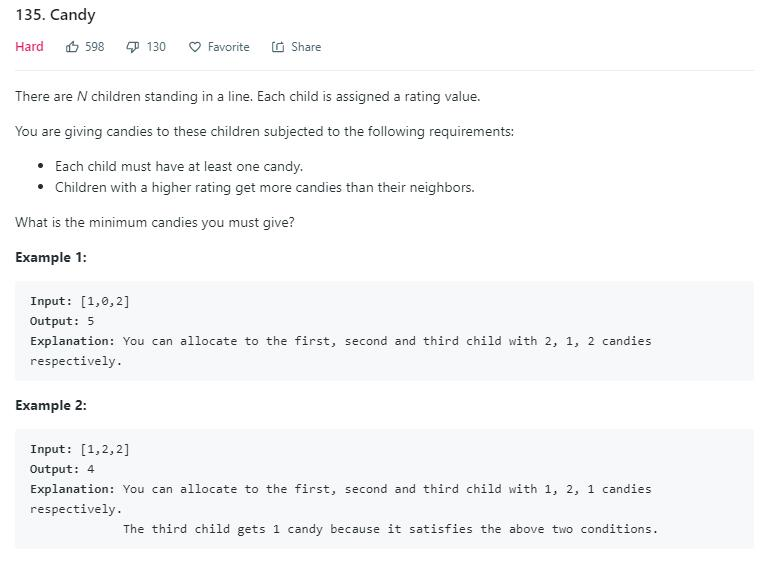- 表示糖，举几个例子。

1 0 2
- - -
-   -

1 2 2
- - -
-



# 解法一

初始化每人一个糖
1 2 3 2 1 4
- - - - - -.

1 < 2
1 2 3 2 1 4
- - - - - -
-

2 < 3
1 2 3 2 1 4
- - - - - -
- -
-

3 > 2 不考虑

2 > 1 不考虑

1 < 4
1 2 3 2 1 4
- - - - - -
- -     -
-

4 1 2 3 2 1
- - - - - -
-     - -
-

4 > 1 不考虑

1 < 2
4 1 2 3 2 1
- - - - - -
-   - - -
-

2 < 3，3 的糖果已经比 2 的多了，不需要考虑

3 > 2，不考虑

2 > 1，不考虑



public int candy(int[] ratings) {
int n = ratings.length;
int[] candies = new int[n];
//每人发一个糖
for (int i = 0; i < n; i++) {
candies[i] = 1;
}
//正着进行
for (int i = 0; i < n - 1; i++) {
//当前小朋友的 rating 比后一个小朋友的小,后一个小朋友的糖是当前小朋友的糖加 1。
if (ratings[i] < ratings[i + 1]) {
candies[i + 1] = candies[i] + 1;
}
}
//倒着进行
//下标顺序就变成了 i i-1 i-2 i-3 ... 0
//当前就是第 i 个，后一个就是第 i - 1 个
for (int i = n - 1; i > 0; i--) {
//当前小朋友的 rating 比后一个小朋友的小
if (ratings[i] < ratings[i - 1]) {
//后一个小朋友的糖果树没有前一个的多，就更新后一个等于前一个加 1
if (candies[i - 1] <= candies[i]) {
candies[i - 1] = candies[i] + 1;
}

}
}
//计算糖果总和
int sum = 0;
for (int i = 0; i < n; i++) {
sum += candies[i];
}
return sum;
}


# 解法二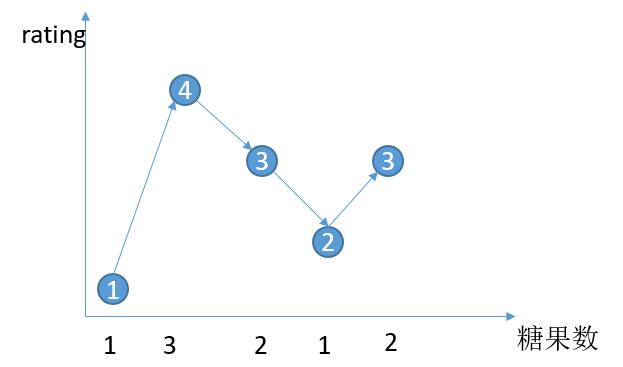1. 左边山底到山顶的高度大，并且右边山底后继续增加。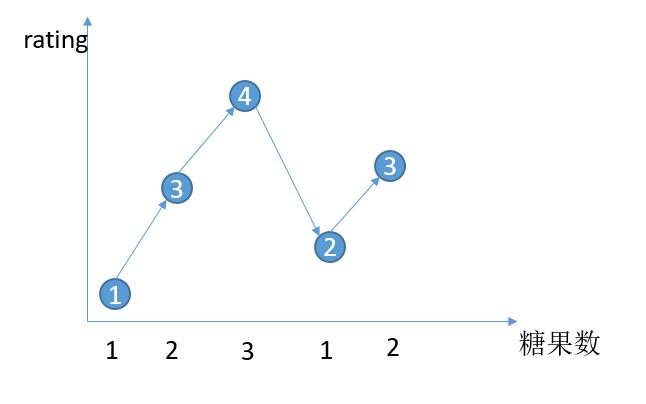2. 左边山底到山顶的高度大，并且右边山底是平坡。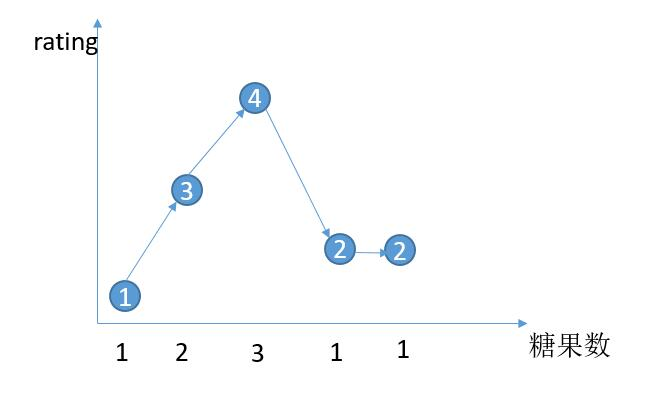3. 右边山底到山顶的高度大，并且右边山底后继续增加。4. 右边山底到山顶的高度大，并且右边山底是平坡。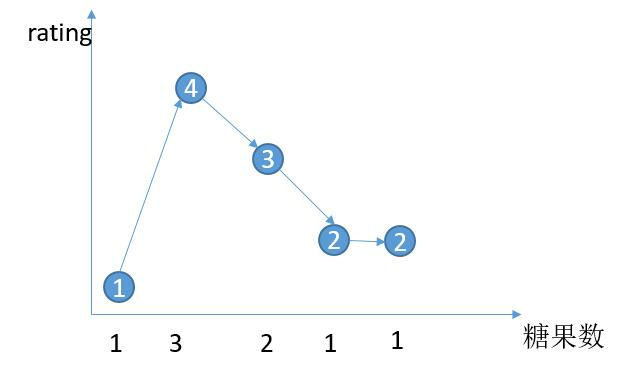public int candy(int[] ratings) {
int n = ratings.length;
int total = 0;
int down = 0;
int pre = 1;
for (int i = 1; i < n; i++) {
//当前是在上坡或者平坡
if (ratings[i] >= ratings[i - 1]) {
//之前出现过了下坡
if (down > 0) {
//山顶的糖果数大于下降的高度，对应情况 1
//将下降的糖果数利用等差公式计算，单独加上山顶
if (pre > down) {
total += count(down);
total += pre;
//山顶的糖果数小于下降的高度，对应情况 3，
//将山顶也按照等差公式直接计算进去累加
} else {
total += count(down + 1);
}

//当前是上坡，对应情况 1 或者 3
//更新 pre 等于 2
if (ratings[i] > ratings[i - 1]) {
pre = 2;

//当前是平坡，对应情况 2 或者 4
//更新 pre 等于 1
} else {
pre = 1;
}
down = 0;
//之前没有出现过下坡
} else {
//将前一个小朋友的糖果数相加
total += pre;
//如果是上坡更新当前糖果数是上一个的加 1
if (ratings[i] > ratings[i - 1]) {
pre = pre + 1;
//如果是平坡，更新当前糖果数为 1
} else {
pre = 1;
}

}
} else {
down++;
}
}
//判断是否有下坡
if (down > 0) {
//和之前的逻辑一样进行相加
if (pre > down) {
total += count(down);
total += pre;
} else {
total += count(down + 1);
}
//将最后一个小朋友的糖果计算
} else {
total += pre;
}
}

//等差数列求和
private int count(int n) {
return (1 + n) * n / 2;
}